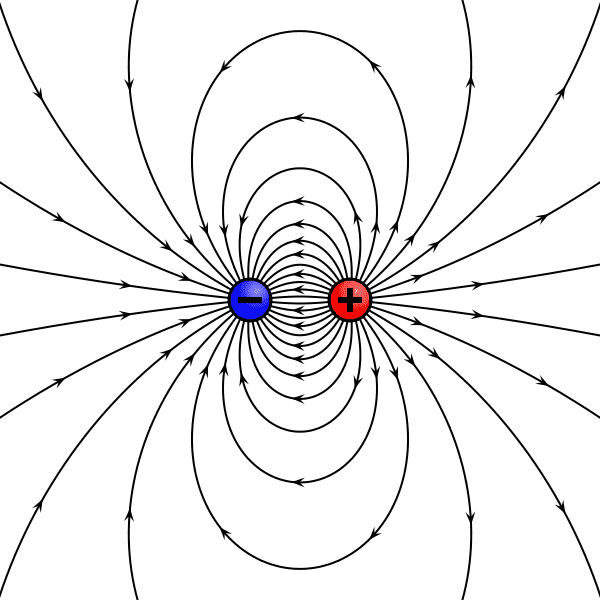# Electric field of AC source

• Artyman

#### Artyman

Hello everybody
I got really confused. I want to calculate the Electric-Field of an alternating current source( Sine(wt)). I do not know what is its equation. I need to run it in Matlab to get a result. Can you please give me some hints?
I need to write the equation to calculate the E-field in different points of a particular distances.
Thanks

AC analysis is about averages for an integer number of cycles. The average of V and I and E and B over complete cycle are zero. So your question makes no sense that way.

Here's how to express it. You have a collection of charges moving in 3D space (say following a wire). Let's say some function f(x,y,z). You can use Maxwell's equations to find the instantaneous field at some other point, say at E at point X1,X2,X3. Now let f vary sinusoidally with time, f(x,y,z,t) at the remote point you will get time dependent results E(t) at point X1, X2, X3. You can sample it with N discrete points for one cycle. So if you want the E field at 100 points, 100 times per cycle, for one whole cycle, then you will get 10000 numbers.

For arbitrarily shaped wires and pieces of iron (such as in a transformer), finite element analysis is sometimes used.

•cnh1995 and Artyman

AC analysis is about averages for an integer number of cycles. The average of V and I and E and B over complete cycle are zero. So your question makes no sense that way.

Here's how to express it. You have a collection of charges moving in 3D space (say following a wire). Let's say some function f(x,y,z). You can use Maxwell's equations to find the instantaneous field at some other point, say at E at point X1,X2,X3. Now let f vary sinusoidally with time, f(x,y,z,t) at the remote point you will get time dependent results E(t) at point X1, X2, X3. You can sample it with N discrete points for one cycle. So if you want the E field at 100 points, 100 times per cycle, for one whole cycle, then you will get 10000 numbers.

For arbitrarily shaped wires and pieces of iron (such as in a transformer), finite element analysis is sometimes used.
My problem is I have sine source( EXP: sin(w1t)), I want to calculate the Electric field ,the current source can create at the point (EXP: P we can assume just having the dielectric at the desire point) ,in a distance from it. I read a lot about it. But, after all reading I got confused. If we have a point charge the electric field in the r distance from can be calculated very easily. But when an alternating current replaces this DC source how this E-field can be calculated( the equation) will be?
Thanks

You don't have a point charge. You have charges flowing in a closed circuit that is distributed in 3D space. An AC source is just a time varying DC source.

•jim hardy
My problem is I have sine source( EXP: sin(w1t)), I want to calculate the Electric field ,the current source can create at the point (EXP: P we can assume just having the dielectric at the desire point) ,in a distance from it.
Well if your sine voltage or current source is driving a capacitor, calculating the E-field inside the capacitor as a function of the capacitor voltage is easy, right? Do you know the equation for that?

And if you have a more complicated geometry than just a parallel-plate capacitor, calculating the E-field between the 2 conductors generating the field usually involves integrals, and sometimes you need to use numerical modeling methods to calculate it (like with COMSOL). Are you familiar with those integrals, and maybe with simulation software packages?•Artyman and dlgoff
Well if your sine voltage or current source is driving a capacitor, calculating the E-field inside the capacitor as a function of the capacitor voltage is easy, right? Do you know the equation for that?

And if you have a more complicated geometry than just a parallel-plate capacitor, calculating the E-field between the 2 conductors generating the field usually involves integrals, and sometimes you need to use numerical modeling methods to calculate it (like with COMSOL). Are you familiar with those integrals, and maybe with simulation software packages?

View attachment 208016
I can understand the whole story...But I do not know how to make a relation between them.
How can I consider timing agent in calculating the E-filed. When it comes to Alternating Current. I do not know to which direction I should go.
Maybe a link or paper even old one may help me.

Maybe it is better to step back a bit. What are you trying to
accomplish?

You're going to have equations with time as a variable.

For the ordinary case of household AC current, sine wave,
Alternating current is Ipeak X sin( ωt )
where Ipeak is maximum instantaneous value of current
and ω = frequency X 2π
and t of course is time.

Now the electric field due to a current is a tough question
because we ordinarily just compute the magnetic field B arising from a current
http://hyperphysics.phy-astr.gsu.edu/hbase/magnetic/amplaw.html
if that current is f(time) so will be the field.

You'll have to go to Maxwell's equations
which tell us every B field has an accompanying E field

I'll not diss Maxwell by trying to explain him . I'm an ant and he's a Lion.

old jim

Maybe it is better to step back a bit. What are you trying to
accomplish?
Just to see what Electric field from AC source at each point of the specific space with (epsilonr= relative permittivity and finite conduction) in X and Y scanning.